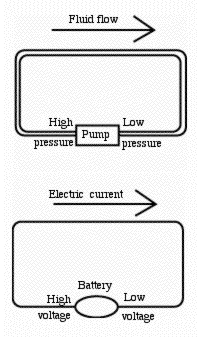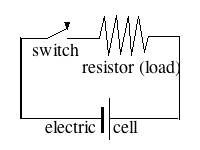# (E2)   Voltage and Ohm's Law

Like water in pipes, electric current is driven by a kind of pressure--we will call it by its common name of voltage, because its units are named volts, after the Italian Alessandro Volta. Volta was the one who in 1800 produced the first "electric battery"; before that the Italian Luigi Galvani produced an electric current chemically in an "electrical cell" in which two different metals dipped into a water solution containing acid. Volta stacked many cells on top of each other to produce a "voltaic pile"; a pile of 20 cells gave 20 times the voltage of a single one--and the current also increased proportionally. The word "battery" originally meant a collection of more than one item of the same kind (battery of cannons, battery of tests), but nowadays it is mainly used for sources of electricity.You measure voltage with a "voltmeter," an instrument with a dial or a read-out panel, and two connections--one to be connected to the "high voltage" side of the circuit, the other to the "low voltage" side (like the high pressure and low pressure between which a pipe carries a flow of water). A physicist might prefer "high electric potential" and "low electric potential," but "voltage" is always understood, and is the term electricians use.

A "dry cell" used in batteries is often a cylinder with a little metal cap on top and a metal body, insulated from the cap. A voltmeter will measure about 1.5 volts between the two--with the higher voltage (the more positive one) at the cap. A typical car's battery has 6 cells with fluid acid sealed in, and gives up to 12 volts, depending on where it stands between "full" and "empty" (and less when a large current is being withdrawn, which is why a car's lights may dim when the starter motor is running).

All electric cells contain a fluid (which may be acid or alkaline) bridging the gap between the cap and the body. Even a so-called "dry" cell contains fluid, but there it is soaked into a substance which stops it from spilling or sloshing. The average voltage driving electricity from a wall outlet in the US is 110 volts (220 volts in Europe), but note this is rapidly changing "alternating current" (AC), a type discussed later.

Now for the formulas.

Just as the flow rate F of water in a pipe (in our simple analogy) may be proportional to the pressure P driving it, so... ...the electric current I in a wire is proportional to the voltage V driving it (at least for copper wires). For the flow of water in pipes we wrote

F = P S     or     F = P / R     ( S = 1 / R )

and the analogous relations for the electric current are

I = V S     or     I = V / R     ( S = 1 / R )The form
I = V / R

is the celebrated "Ohm's law," named after the German Georg Ohm who proposed it. When I is measured in amperes and V in volts, the units of the electrical resistance R are known as ohms. A voltage of 1 volt, faced with a resistance of one ohm, will drive through it a current of one ampere.

(To prepare the ground, the word "ohm" was earlier slipped into the formula F = P/R for resistance of water pipes, although it really does not belong there. However the term "electric conductance" is a valid one for S = 1/R. In I = V S , if I is measured in amperes and V in volts, then the unit of S is officially named the "Siemens", and unofficially--what else?--"mho," pronounced like "moh" or "mhoh.")

Please note: The simple proportionality expressed by Ohm's law is a useful approximation to what is observed in metal wires, but more complicated relations may exist. It is not a fundamental law of electricity, but a useful approximation to the electric behavior of conductors. One notable exception is the plasma in a fluorescent light source.

### To remember

 "Voltage" is the electric counterpart to pressure. Just as the water flow F through a pipe may be proportional (in our analogy) to the pressure difference between its ends, so the electric current I through a wire is proportional to the voltage between its ends. Also, as water flows from high pressure to lower one, so electricity flows from the higher positive voltage to the lower one, or to where it is negative or zero.     Ohm's law says that the current I is inversely proportional to the resistance of a wire, which like that of a pipe increases with length and decreases with growing diameter (it also depends on the conducting substance). Expressing this in a formula I = V / R S =1/R is the "conductance" of the wire, also used sometimes, and I = V S.

Next Stop: E3.   Electric Circuits

"From Stargazers to Starships"
Timeline of "All Things Electric and Magnetic"
Timeline (from "Stargazers")
Master index file

Author and Curator:   Dr. David P. Stern
Mail to Dr.Stern:   stargaze("at" symbol)phy6.org .

Posted 17 September 2009